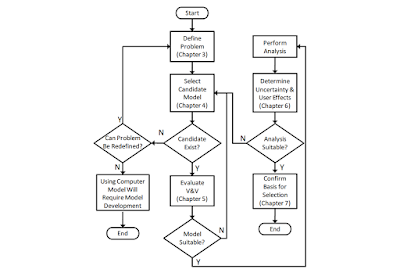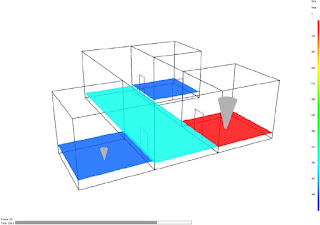### An Introduction to Fire Modelling

"Of critical importance when using models for Fire Safety Engineering is that the user knows the limitations and the appropriateness of the specific model being used. The misuse/incorrect use could have great consequences; it could be that a life safety system (for example a smoke control system) is designed using a tool that is not appropriate for that purpose. This could lead to an incorrect design, with a possible result being a reduced level of safety for the occupants – particularly if an under-engineered solution results in a system unable to deal with the actual situation, such as more smoke production than a ventilation system was designed to manage because the design tool used was not appropriate for that situation. Engineers are often responsible for the life safety analysis, and we are equipped with a number of tools to aid the design process. One challenge is to understand how to use such tools, but of equal and paramount importance is understanding their limitations, and thus when their use is not appropriate."

##Introduction

There is a wide variety of models available for the prediction of a various fire phenomena. These range from simple Algebraic correlations that can be solved with a calculator, to Zone Models or Lumped Parameter Models, which represent a space as a small number of elements, to Computational Fluid Dynamics (CFD) or Field Models, which approximate a space as a large number of discrete volumes. In general, Zone Models are more sophisticated than Algebraic correlations, and CFD models are more sophisticated than Zone Models.

In all types of design situations a number of factors should be considered before selecting a particular model for a problem, including computational resources, time limitations, required level of accuracy, and most importantly, whether or not the governing equations and assumptions in the model are appropriate for the problem of interest. However, increased sophistication does not always translate into better results, as in many cases a simple model will be sufficient, or insufficient data will be available for the use of a more sophisticated model. For a given application, there may be one, multiple, or no fire models available.

## Algebraic Models

Algebraic models are generally the first step employed during a fire dynamics analysis. Equations have been developed to estimate the behaviour of fire effects such as flame height, heat release rate, plume and ceiling jet velocities, gas layer temperatures and depth, radiation, and other phenomena.

Algebraic models employ equations that have been developed either empirically using observations from fire test data or mathematically through direct calculation of physical properties and behaviours. These equations often yield steady state results, providing information at a moment in time; however, many can also be iterated to provide transient data by solving the equation(s) over a number of time steps.

The benefits of algebraic models are that they can provide an immediate answer to simple fire dynamics questions and basic fire phenomena and that the results of these equations are directly based on empirical data from testing or fundamental physics. The drawbacks of algebraic models are that they are often tailored for specific simplified scenarios and that they generally provide information about a single property of interest, rather than a more detailed representation of a fire’s behaviour as a whole. The correlation’s predictive capability will most closely match the actual conditions when the scenario most closely matches the test conditions from which the correlation came. The greater the differences between the scenario and the original test conditions the lesser the confidence in the predictive answer of the correlation.

Even in cases where algebraic models are not chosen as the final method of analysis, it is often useful to use these simple calculations to provide a check of the output obtained from more complicated forms of modelling. Although the solutions to these models may not exactly match the output from a zone model or computational fluid dynamics model, they are often of the same order of magnitude as the results provided by the more complex models. If the results of an algebraic model and another type of model are significantly different, further analysis should be conducted to determine why this is and whether or not it is representative of an error in part of the analysis that should be corrected. The drawbacks of algebraic models are that they are often tailored for specific simplified scenarios and that they generally provide information about a single property of interest, rather than a more detailed representation of a fire’s behavior as a whole. The correlation’s predictive capability will most closely match the actual conditions when the scenario most closely matches the test conditions from which the correlation came. The greater the differences between the scenario and the original test conditions the lesser the confidence in the predictive answer of the correlation.

##Zone Models

When an algebraic equation does not provide enough detail or accuracy to determine the fire phenomena of interest, the next step is often to use a zone model or lumped parameter model.

Zone models and lumped parameter models operate under the assumption that a given space can be broken into a small number of volumes, or “zones”, over which a number of algebraic equations are solved to determine the relevant flow properties.

For the purposes of computer fire modelling, these models generally consider two or three zones, including the lower layer, the upper layer and sometimes a zone used to represent the plume or ceiling jet in the compartment with the fire. Temperatures, velocities and other properties are assumed to be uniform within these zones; the transfer of mass, energy, momentum and species are tracked from one zone to another via equations that have been tailored for the “zone assumption”.

The two-layer approach to fire zone models is based on the phenomena that hot gases will collect at the ceiling of a compartment while a cooler layer will be present below the hot gasses. Although experimental results indicate that there are in reality variations in conditions across these zones, they have been found to be smaller than the differences between the layers, thereby allowing a realistic simulation of many common fire conditions using zone models. As such, zone models are often employed in order to track properties associated with the transport of smoke and hot gases from the compartment of fire origin to other portions of buildings via connected volumes. The outputs of zone models depend upon a user-defined characterization of the design fire.

With advances in the capabilities of personal computers, it has become possible to run zone models of multi-compartment fire scenarios in a matter of seconds or minutes. This allows fire modellers to obtain more information than might be available through the use of algebraic models while still being able to test a wide range of variables that may affect the behaviour of a fire. The drawbacks of the zone model are that there are times when the assumption of uniform zones is not accurate, and that it is difficult (or at times impossible) to model spaces that have complex geometries that are not accurately simulated using equivalent rectangular volumes. Similarly, some zone model assumptions, such as the movement of hot gases to the ceiling, may not be true under a scenario with a large ceiling height where stratification occurs.

Some zone models have additional sub-models to simulate specific phenomena not inherently consistent with the zone assumption. For example, some zone models simulate detector or sprinkler actuation through the use of ceiling jet calculations. They may calculate the velocity and temperature within a fire room, but assume a velocity, outside the fire room, as the result of a door plume. In the case of sprinkler actuation, the two-zone assumption breaks down when the sprinkler actuates. While this may be adequately covered through suppression correlations when a single sprinkler operates, such an approach does not currently work for multiple sprinklers simultaneously operating.

## Field Models

Field models, or computational fluid dynamics (CFD) models, provide a method for modelling the fluid flow through a volume using numerical solutions of the Navier-Stokes equations. The solutions of partial differential equations for conservation of mass, momentum and energy are approximated as finite differences over a number of control volumes. In most CFD software packages, these calculations can be performed over a period of time to provide a transient solution. Rather than using two or three control volumes as with a zone model, a CFD model is typically comprised of hundreds of thousands (or millions) of control volumes. This achieves a finer level of resolution than models that utilize the zone assumption, but at the cost of requiring greater computational resources and extended simulation times of days, weeks or even months.

##In addition to addressing fluid phenomena such as turbulence and buoyancy, the use of CFD models to simulate a fire-driven flow should also account for physical phenomena such as the spread of hot gasses, thermal radiation, and tracking of particles of smoke and water.

Benefits of CFD models include their basis in the fundamental physics of fluid flow, a flexibility that allows the simulation of a large range of boundary conditions, and the ability to model much more detailed geometries than can be achieved using algebraic or zone models. Drawbacks of CFD models include increased complexity, elevated need for training of the model user, greater computational requirements, and a longer timeline between initiating a project and completing the necessary simulations. The most advanced and sophisticated fire modelling technique is the use of Computational Fluid Dynamics (CFD) models to predict fire growth and compartment temperatures. CFD models have been shown to be successful in the modelling of smoke movement and have recently been applied to the modelling of fires. They are capable of modelling pre-flashover and localised fires in complex geometries with smoke movement in multi-compartments.

Typical CFD models analyse systems involving fluid flow, heat transfer and associated phenomena by solving the fundamental equations of the fluid flow. These equations represent the mathematical statements of the conservation laws of physics:
the mass of a fluid is conserved;
the rate of change of momentum equals the sum of the forces on a fluid particle – the Newton’s second law;
the rate of change of energy is equal to the sum of the rate of heat increase and the rate of work done on a fluid particle – the first law of thermodynamics.

Basically, in a CFD model, the partial differential equations of the thermodynamic and aerodynamic variables (Navier-Stokes equations) are solved in a very large number of points in the compartments. Most CFD models for enclosure fires are appropriate for low-speed, thermally-driven flow with an emphasis on smoke and heat transport from fires.